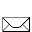A b d u c c i o n e s   E x t r a t e r r e s t r e s  por   J o h n   M a c k   (  Documental en Español  )   SAQUE USTED MISMO, SUS PROPIAS CONCLUSIONES....   P A R T E   IP A R T E   IIP A R T E   IIIP A R T E   IVP A R T E   VP A R T E   VIinfo@erks.org

 www.erks.org  -  I N F O R M A C I Ó N   C L A S I F I C A D A